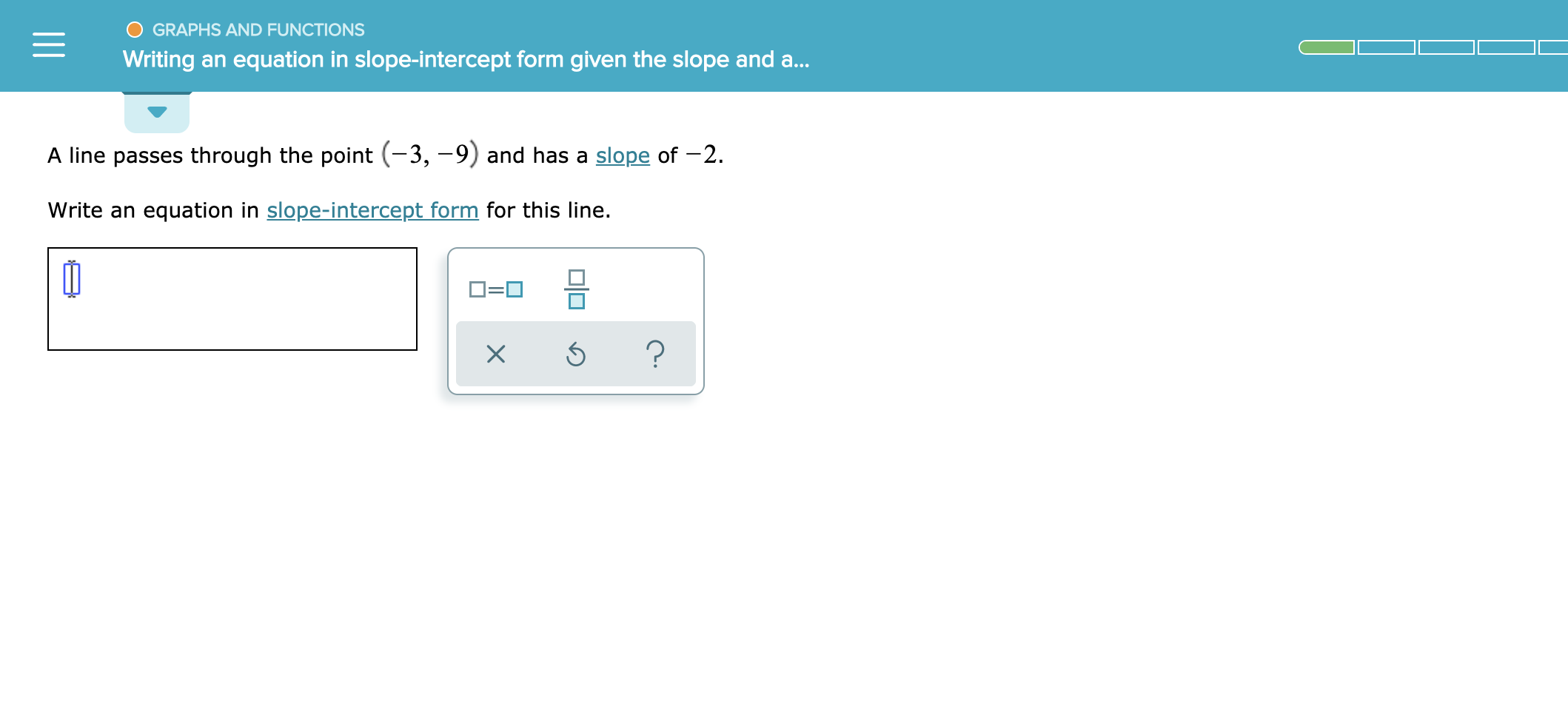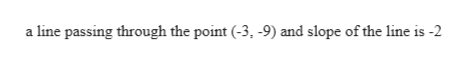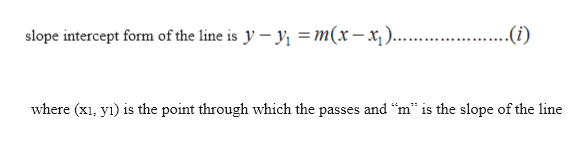GRAPHS AND FUNCTIONSWriting an equation in slope-intercept form given the slope and a..A line passes through the point (-3, -9) and has a slope of -2.Write an equation in slope-intercept form for this line.?X

Question

See attachedhelp_outlineImage TranscriptioncloseGRAPHS AND FUNCTIONS Writing an equation in slope-intercept form given the slope and a.. A line passes through the point (-3, -9) and has a slope of -2. Write an equation in slope-intercept form for this line. ? X fullscreen
Step 1

given thathelp_outlineImage Transcriptionclosea line passing through the point (-3, -9) and slope of the line is -2 fullscreen
Step 2

Slope-intercept form ...help_outlineImage Transcriptioncloseslope intercept form of the line is y y m(x-x.. .(i) where (x y) is the point through which the passes and "m" is the slope of the line fullscreen

Want to see the full answer?

See Solution

Want to see this answer and more?

Our solutions are written by experts, many with advanced degrees, and available 24/7

See Solution
Tagged in

Other